# Texas Go Math Grade 5 Lesson 6.2 Answer Key Multiply Fractions and Whole Numbers

Refer to our Texas Go Math Grade 5 Answer Key Pdf to score good marks in the exams. Test yourself by practicing the problems from Texas Go Math Grade 5 Lesson 6.2 Answer Key Multiply Fractions and Whole Numbers.

## Texas Go Math Grade 5 Lesson 6.2 Answer Key Multiply Fractions and Whole Numbers

Investigate

Martin is planting a vegetable garden. Each row is two meters long. He wants to plant carrots along $$\frac{3}{4}$$ of each row. How many meters of each row will he plant with carrots?

Multiply. $$\frac{3}{4}$$ × 2
Materials; fraction strips; MathBoard

A. Place two 1-whole fraction strips side-by-side to represent the length of the garden.

B. Find 4 fraction strips all with the same denominator that fit exactly under the two wholes.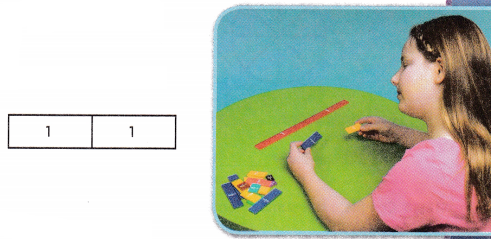C. Draw a picture of your model. ____________________

D. Circle $$\frac{3}{4}$$ of 2 on the model you drew.

E. Complete the number sentence. $$\frac{3}{4}$$ × 2 = _________
So, Martin will plant carrots along _________ meters of each row.

Multiply (3/4) × 2
A. Place two 1-whole fraction strips side-by-side to represent the length of the garden.
B. Find 4 fraction strips all with the same denominator that fit exactly under the two wholes.
C. Draw a picture of our model.D. Circle 3/4 of 2 on the model we drew.
E. Complete the number sentence.
(3/4) × 2 = 6/4 = 3/2 or 1(1/2)
So, Martin will plant carrots along 1(1/2) meters of each row.

Draw Conclusions

Question 1.
Explain why you placed four fraction strips with me the same denominator under the two 1-whole strips.
I wanted to divide the entire length of the two wholes into four equal parts. The 4, 1/2 fractions strips did that.

Question 2.
Explain how you would model $$\frac{3}{10}$$ of 2?
I could divide the two wholes into ten equal parts using 10, 1/5 fraction strips. I would circle 3/10 of the 1/5 strips. This would equal 3/5.

Make Connections

You can also use a model to multiply a fraction by a whole number.

Margo was helping clean up after a class party. There were 3 boxes remaining with pizza in them. Each box had $$\frac{3}{8}$$ of a pizza left. How much pizza was left in all?
Materials; fraction circles
STEP 1: Find 3 × $$\frac{3}{8}$$. Model three 1-whole fraction circles to represent the number of boxes containing pizza.

STEP 2: Place $$\frac{1}{8}$$ fraction circle pieces on each circle to represent the amount of pizza that was left in each box. Shade the fraction circles below to show your model.Each circle shows ________ eighths of a whole.
The 3 circles show ________ eighths of a whole.

STEP 3: Complete the number sentences.
$$\frac{3}{8}$$ + $$\frac{3}{8}$$ + $$\frac{3}{8}$$ = ____________
3 × $$\frac{3}{8}$$ = ____________
So, Margo had __________ boxes of pizza left.
STEP 1: Find 3 × 3/8. Model three 1-whole fraction circles to represent the number of boxes containing pizza.
STEP 2: Place 1/8 fraction circle pieces on each circle to represent the amount of pizza that was left in each box. Shade the fraction circles below to show your model.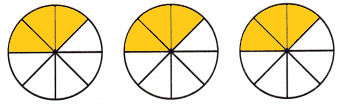Each circle shows 3 eighths of a whole.
The 3 circles show 9 eighths of a whole.
STEP 3: Complete the number sentences.
3/8 + 3/8 + 3/8 = 9/8
3 × 3/8 = 9/8
So, Margo had 9/8 or 1(1/8) boxes of pizza left.

Math Talk
Mathematical Processes

Explain how you would know there is more than one pizza left.

Share and Show

Use the model to find the product.

Question 1.
$$\frac{5}{6}$$ × 3 = __________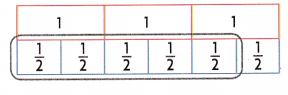5/6 x 3 = 15/6 = 5/2 or 2(1/2)
Explanation:
In the above image we can observe three 1-whole fraction strips side-by-side. The 6 fraction strips all with the same denominator that fit exactly under the three wholes. So, drawn a circle for 5/2 on the model given. The number sentence is (5/6) × 3 = 5/2.

Question 2.
2 × $$\frac{5}{6}$$ = ____________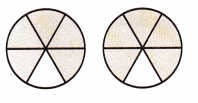2 x (5/6) = 10/6 = 5/3 or 1(2/3)
Explanation:
In the above image we can observe two circles. Each circle shows 5 six’s of a whole. The 2 circles show 10 six’s of a whole. The number sentence is 2 × 5/6 = 10/6 = 5/3 or 1(2/3).

Problem Solving

Pose a Problem

Question 3.
Tarique drew the model below for a problem. Write 2 problems that can be solved using this model. One of your problems should involve multiplying a whole number by a fraction and the other problem should involve multiplying a fraction by a whole number.Pose a problem.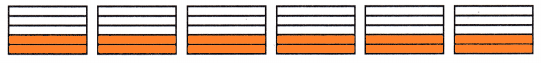Explanation:
Pose a problem:
1. A gardener is planting flowers in 6 rows of the garden. He will plant 2/5 of the 6 rows with 6 roses. How many rows will be filled with roses.
2. A gardener planted 2/5 of a row with roses. If he plants 5 more rows like first row, how many rows of roses will there be. When all the 6 rows are planted.
1. (2/5) of 6 = (2/5) x 6 = 12/5 or 2(2/5)
2(2/5) rows will be roses.
2. 6 x (2/5) = 12/5 or 2 (2/5)
2(2/5) rows will be roses.

Question 4.
H.O.T. Multi-Step How could you change the model to give you an answer of 4$$\frac{4}{5}$$? Explain and write a new equation.6 x (4/5) = 24/5 or 4(4/5)
Explanation:
In the above image we can observe 6 rectangles. I change the model to give an answer of 4(4/5). I would shade 2 more sections in each rectangle to get 24/5 or 4(4/5).

Question 5.
Carly mixes vinegar and baking soda for a science project. She has a spoon that measures $$\frac{1}{4}$$ teaspoon. If she fills the spoon 6 times, how much baking soda will she have?
(A) $$\frac{1}{10}$$ teaspoon
(B) $$\frac{2}{3}$$ teaspoon
(C) 1$$\frac{1}{2}$$ teaspoons
(D) 1$$\frac{3}{4}$$ teaspoons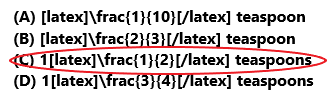6 x (1/4) = 3/2 = 1(1/2)
Carly have 1(1/2) baking soda.
So, option C is correct.
Explanation:
Carly mixes vinegar and baking soda for a science project. She has a spoon that measures 1/4 teaspoon. She fills the spoon 6 times. Multiply 6 with 1/4 the product is 3/2. The fraction 3/2 in mixed fraction form is 1(1/2). Carly have 1(1/2) baking soda. So, draw a circle to option C.

Question 6.
Use Tools Which multiplication problem does the model represent?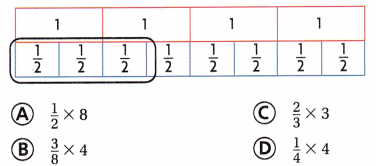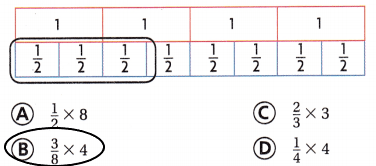3/8 x 4 = 3/2
So, option B is correct.
Explanation:
In the above image we can observe four 1-whole fraction strips side-by-side. The 8 fraction strips all with the same denominator that fit exactly under the four wholes. So, drawn a circle for 3/8 of 4 on the model given. The number sentence is (3/8) × 4 = 3/2. So, the multiplication (3/8) x 4 represents the above model.

Question 7.
Multi-Step Josh brought 4 small spinach pies to his baseball team party. At the end of the party, $$\frac{3}{5}$$ of each pie was left. If Josh gave 2 whole pies away, what part of a pie did he have left to take home?
(A) $$\frac{2}{5}$$
(B) $$\frac{5}{6}$$
(C) $$\frac{1}{3}$$
(D) $$\frac{1}{2}$$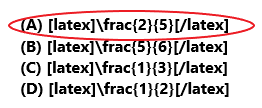4 x (3/5) = 12/5
(12/5) – 2 = 2/5
Josh have 2/5 part of a pie left to take home.
So, option A is correct.
Explanation:
Josh brought 4 small spinach pies to his baseball team party. At the end of the party, 3/5 of each pie was left. Multiply 4 with 3/5 the product is 12/5. Josh gave 2 whole pies away. Subtract 2 from 12/5 the difference is 2/5. Josh have 2/5 part of a pie left to take home. So, draw a circle to option A.

Texas Test Prep

Question 8.
Katana has a shelf that is 5 feet long. She wants to paint a design along $$\frac{7}{10}$$ of the shelf. How many feet of the shelf will Katana paint a design?
(A) 1$$\frac{2}{5}$$ feet
(B) 1$$\frac{1}{5}$$ feet
(C) 3$$\frac{1}{2}$$ feet
(D) 4$$\frac{3}{10}$$ feet5 x (7/10) = 7/2 = 3(1/2)
Katana paint a design 3(1/2) feet of the shelf.
So, option C is correct.
Explanation:
Katana has a shelf that is 5 feet long. She wants to paint a design along 7/10 of the shelf. Multiply 5 with 7/10 the product is 7/2. The fraction 7/2 in mixed fraction form is 3(1/2). Katana paint a design 3(1/2) feet of the shelf.
So, draw a circle to option C.

### Texas Go Math Grade 5 Lesson 6.1 Homework and Practice Answer Key

Use the model to find the product.

Question 1.Explanation:
In the above we can observe two 1-whole fraction strips side-by-side. The 10 fraction strips all with the same denominator that fit exactly under the two wholes. So, circle is already drawn for (9/10) x 2 on the model given. The number sentence is (9/10) × 2 = 9/5.

Question 2.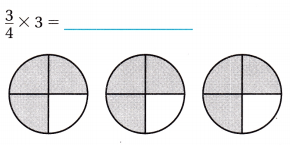Explanation:
In the above image we can observe three circles. Each circle is shaded 3 parts out of 4. The 3 circles are shaded 9 parts out of 12. The number sentence is (3/4) x 3 = 9/4.

Question 3.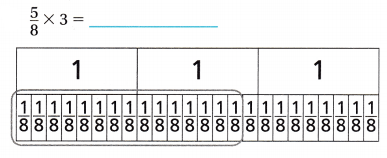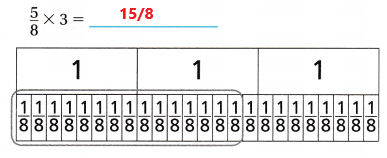Explanation:
In the above we can observe three 1-whole fraction strips side-by-side. The 24 fraction strips all with the same denominator that fit exactly under the three wholes. So, circle is already drawn for (5/8) x 3 on the model given. The number sentence is (5/8) × 3 = 15/8.

Question 4.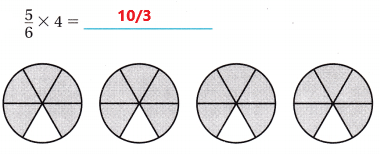Explanation:
In the above image we can observe four circles. Each circle is shaded 5 parts out of 6. The 4 circles are shaded 20 parts out of 24. The number sentence is (5/6) x 4 = 10/3.

Question 5.Explanation:
In the above we can observe two 1-whole fraction strips side-by-side. The 12 fraction strips all with the same denominator that fit exactly under the two wholes. So, circle is already drawn for (7/12) x 2 on the model given. The number sentence is (7/12) × 2 = 7/6.

Question 6.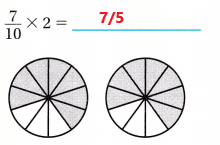Explanation:
In the above image we can observe two circles. Each circle is shaded 7 parts out of 10. The 2 circles are shaded 14 parts out of 20. The number sentence is (7/10) x 2 = 7/5.

Problem Solving

Question 7.
Chef Talbot is baking 6 blueberry pies. If he uses 3/4 pint of blueberries in each pie, how many pints of blueberries will he need?
6 x 3/4 = 9/2 = 4(1/2)
He need 4(1/2) pints of blueberries.
Explanation:
Chef Talbot is baking 6 blueberry pies. He uses 3/4 pint of blueberries in each pie. Multiply 6 with 3/4 the product is 9/2. The fraction 9/2 in mixed fraction form is 4(1/2). He need 4(1/2) pints of blueberries.

Question 8.
Mr. McGregor pours $$\frac{3}{8}$$ pound of dirt in each of his 4 flower pots. How much dirt does Mr. McGregor use to fill the 4 pots?
(3/8) x 4 = 12/8 = 3/2 or 1(1/2)
McGregor needs 1(1/2) pounds of dirt to fill the 4 pots.
Explanation:
Mr. McGregor pours 3/8 pound of dirt in each of his 4 flower pots. Multiply 3/8 with 4 the product is 3/2. The fraction 3/2 in mixed fraction form is 1(1/2). McGregor needs 1(1/2) pounds of dirt to fill the 4 pots.

Lesson Check

Question 9.
Which multiplication problem does the model represent?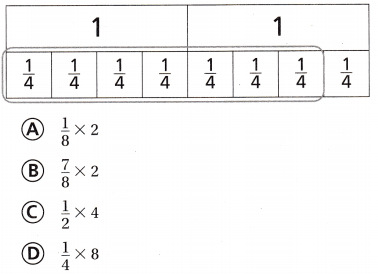7/8 x 2 = 7/4
So, option B is correct.
Explanation:
In the above image we can observe two 1-whole fraction strips side-by-side. The 8 fraction strips all with the same denominator that fit exactly under the two wholes. So, drawn a circle for 7/8 of 2 on the model given. The number sentence is (7/8) × 2 = 7/4. So, the multiplication (7/8) x 2 represents the above model.

Question 10.
Which multiplication problem does the model represent?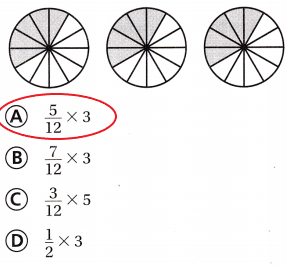Option A is correct.
Explanation:
In the above image we can observe 3 circles. Each circle is shaded with 5 parts out 12 parts. Multiply (5/12) with 3 the product is 5/4. The multiplication problem (5/12) x 3 represents the above model.

Question 11.
Marianne is completing a 4-mile route for charIty Every $$\frac{1}{10}$$ mile is marked along the route. For each mile, she runs $$\frac{7}{10}$$ mile and walks $$\frac{3}{10}$$ mile. How many miles does Marianne run?
(A) 1$$\frac{1}{10}$$ miles
(B) 2$$\frac{4}{5}$$ miles
(C) 1$$\frac{1}{5}$$ miles
(D) 2$$\frac{2}{5}$$ miles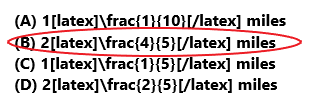4 x (7/10) = 14/5 = 2(4/5)
Marianne runs 2(4/5) miles.
So, option B is correct.
Explanation:
Marianne is completing a 4-mile route for charity. Every 1/10 mile is marked along the route. For each mile, she runs 7/10 mile and walks 3/10 mile. Multiply 4 miles with 7/10 the product is 14/5. The fraction 14/5 in mixed fraction is 2(4/5). Marianne runs 2(4/5) miles. So, draw a circle to option B.

Question 12.
Terrance runs 5 miles each week. His brother runs $$\frac{5}{6}$$ the distance Terrance runs in one week. How far does Terrance’s brother run in one week?
(A) 3$$\frac{1}{3}$$ miles
(B) 4$$\frac{1}{6}$$ miles
(C) 5$$\frac{1}{6}$$ miles
(D) 4 miles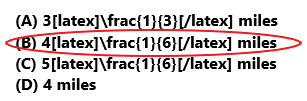5 x (5/6) = 25/6 = 4(1/6)
Terrance’s brother run 4(1/6) miles in one week.
So, option B is correct.
Explanation:
Terrance runs 5 miles each week. His brother runs 5/6 the distance Terrance runs in one week. Multiply 5 miles with 5/6 the product is 25/6. The fraction form of 25/6 in mixed fraction is 4(1/6). Terrance’s brother run 4(1/6) miles in one week. So, draw a circle to option B.

Question 13.
Multi-Step Colton’s recipe makes 2 dozen brownies. His recipe calls for $$\frac{7}{8}$$ cup of vegetable oil. How much oil will Colton need to make 6 dozen brownies?
(A) 2$$\frac{5}{8}$$ cups
(B) 1$$\frac{3}{4}$$ cups
(C) 5$$\frac{1}{4}$$ cups
(D) 3$$\frac{1}{2}$$ cups3 x 7/8 = 21/8 = 2(5/8)
Colton need 2(5/8) cups of oil to make 6 dozen brownies.
So, option A is correct.
Explanation:
Colton’s recipe makes 2 dozen brownies. His recipe calls for 7/8 cup of vegetable oil. Multiply 3 with 7/8 the product is 21/8. The fraction 21/8 in mixed fraction form is 2(5/8). Colton need 2(5/8) cups of oil to make 6 dozen brownies. So, draw a circle to option A.

Question 14.
Multi-Step Kiesha brought 3 loaves of cornbread to a football party. $$\frac{5}{12}$$ of each loaf was eaten. If Kiesha gave 1 whole loaf of the leftover bread to the party hosts, what part of a loaf did she have left to take home?
(A) $$\frac{3}{4}$$
(B) $$\frac{1}{4}$$
(C) $$\frac{1}{2}$$
(D) $$\frac{7}{12}$$